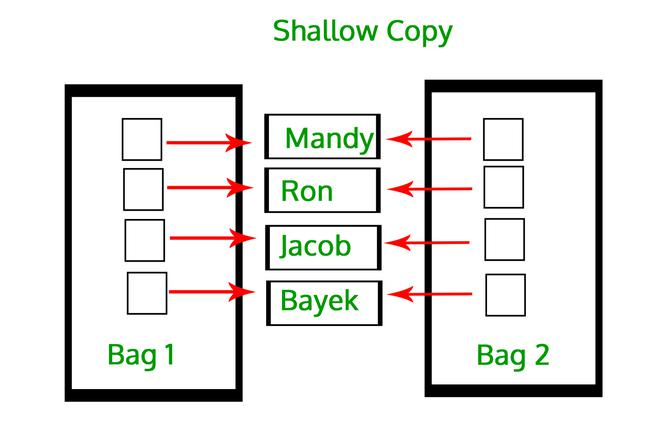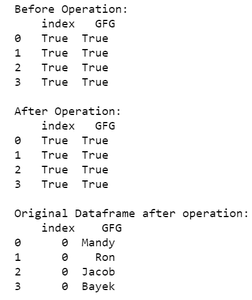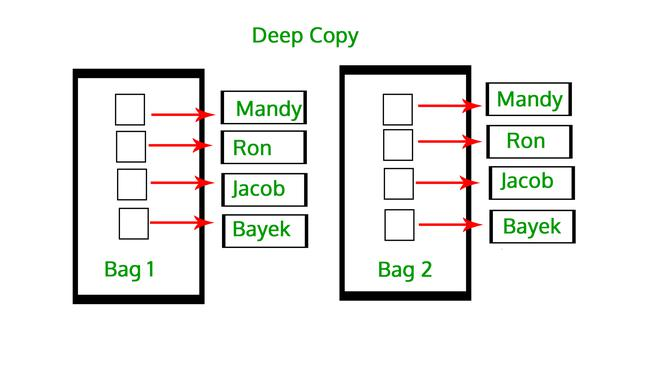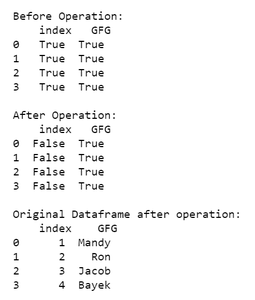# Difference Between Shallow copy VS Deep copy in Pandas Dataframes

• Difficulty Level : Easy
• Last Updated : 09 Feb, 2022

The pandas library has mainly two data structures DataFrames and Series. These data structures are internally represented with index arrays, which label the data, and data arrays, which contain the actual data. Now, when we try to copy these data structures (DataFrames and Series) we essentially copy the object’s indices and data and there are two ways to do so, namely Shallow Copy and Deep Copy.

These operations are done with the help of the library functions pandas.DataFrame.copy(deep=False) for shallow copy and pandas.DataFrame.copy(deep=True) for deep copy in DataFrames and Series.

Now, let’s understand what shallow copying is.

## Shallow Copy

When a shallow copy of a DataFrame or Series object is created, it doesn’t copy the indices and the data of the original object but it simply copies the references to its indices and data. As a result of which, a change made to one is reflected in the other one.

It refers to constructing a new collection object and then populating it with references to the child objects found in the original. The copying process does not recurse and therefore won’t create copies of the child objects themselves.

Example:## Python3

 `# program to depict shallow copy``# in pandas dataframe` `# import module``import` `pandas as pd` `# assign dataframe``df ``=` `pd.DataFrame({``'index'``: [``1``, ``2``, ``3``, ``4``],``                   ``'GFG'``: [``'Mandy'``, ``'Ron'``, ``'Jacob'``, ``'Bayek'``]})`  `# shallow copy``copydf ``=` `df.copy(deep``=``False``)` `# comparing shallow copied dataframe``# and original dataframe``print``(``'\nBefore Operation:\n'``, copydf ``=``=` `df)` `# assignment operation``copydf[``'index'``] ``=` `[``0``, ``0``, ``0``, ``0``]`  `# comparing shallow copied dataframe``# and original dataframe``print``(``'\nAfter Operation:\n'``, copydf ``=``=` `df)` `print``(``'\nOriginal Dataframe after operation:\n'``, df)`

Output:As we can see from the output of the above program, the changes applied to the shallow copied data frame gets automatically applied to the original dataframe.

## Deep Copy

A deep copy of a DataFrame or a Series object has its own copy of index and data. It is a process in which the copying process occurs recursively. It means first constructing a new collection object and then recursively populating it with copies of the child objects found in the original. In the case of deep copy, a copy of an object is copied into another object. It means that any changes made to a copy of the object do not reflect in the original object.

Example:## Python3

 `# program to depict deep copy``# in pandas dataframe` `# import module``import` `pandas as pd` `# assign dataframe``df ``=` `pd.DataFrame({``'index'``: [``1``, ``2``, ``3``, ``4``],``                   ``'GFG'``: [``'Mandy'``, ``'Ron'``, ``'Jacob'``, ``'Bayek'``]})` `# deep copy``copydf ``=` `df.copy(deep``=``True``)` `# comparing shallow copied dataframe``# and original dataframe``print``(``'\nBefore Operation:\n'``, copydf ``=``=` `df)` `# assignment operation``copydf[``'index'``] ``=` `[``0``, ``0``, ``0``, ``0``]`  `# comparing shallow copied dataframe``# and original dataframe``print``(``'\nAfter Operation:\n'``, copydf ``=``=` `df)` `print``(``'\nOriginal Dataframe after operation:\n'``, df)`

Output:Here, the data inside the original objects are not recursively copied. That is, the data inside the data of the original objects still point to the same memory unit. For example, if the data in a Dataframe or Series object contains any mutable data then it will be shared between it and its deep copy and any modification to one will be reflected in the other one.

## Table of Difference Between Shallow Copy V/S Deep Copy

My Personal Notes arrow_drop_up